# ISEE Upper Level Math : How to find the answer from a table

## Example Questions

2 Next →

### Example Question #11 : Tables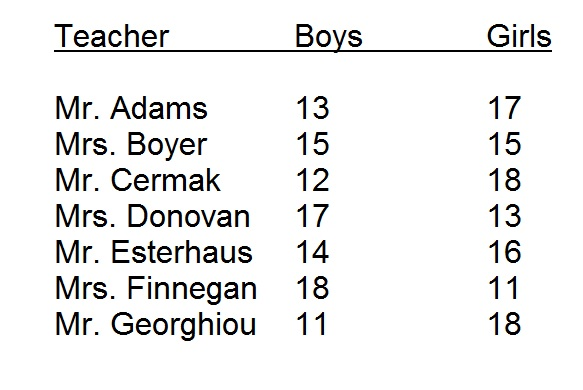Washington Elementary School has seven eighth-grade teachers; each teacher has the number of boys and girls listed above.

An eighth-grade girl has transferred from another school. It is desired that no teacher have more than thirty students overall or eighteen girls in his or her class. Of the seven teachers, how many could accept the girl into his or her class?

Seven

One

Six

Two

One

Explanation:

We can eliminate Cermak and Georghiou immediately since each has eighteen girls in his class. Of the other five:

Adams has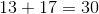students;

Boyer has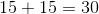students;

Donovan has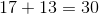students;

Esterhaus has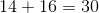students;

Finnegan has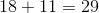students.

Only Mrs. Finnegan's class could accept the new girl without going against the wishes of the school. The correct response is one.

### Example Question #12 : Tables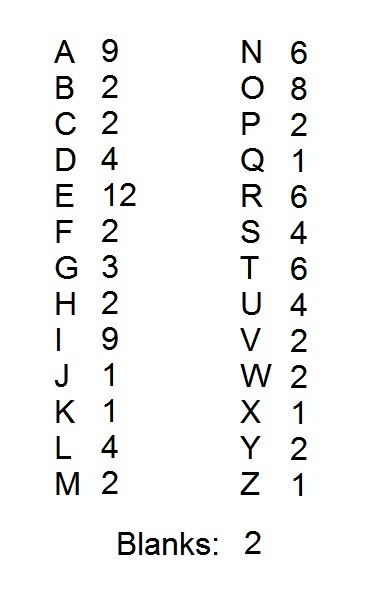A popular word game uses one hundred tiles, each of which is marked with a letter or a blank. The distribution of the tiles is shown above, with each letter paired with the number of tiles marked with that letter. Notice that there are two blank tiles.

What percent of the tiles are marked with consonants?

Note: for purposes of this question, "Y" is a consonant.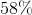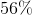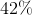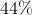Explanation:

Counting the number of tiles with vowels is much easier.

Out of the 100 tiles, there are nine "A" tiles, twelve "E" tiles, nine "I" tiles, eight "O" tiles, and four "U" tiles. This is a total of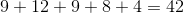tiles out of 100.

There are also two blanks, so the number of tiles marked with consonants is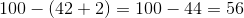56 out of 100 is 56%.

### Example Question #13 : Tables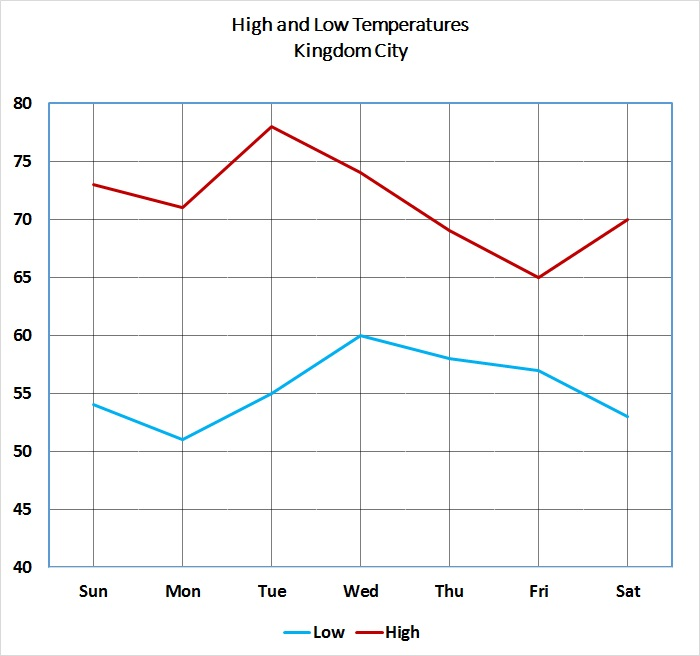Refer to the above graph, which shows the high and low temperatures for Kingdom City over a one-week period.

Between which two consecutive days did the high temperature show an increase?

Between Wednesday and Thursday

Between Tuesday and Wednesday

Between Thursday and Friday

Between Monday and Tuesday

Between Monday and Tuesday

Explanation:

Examining the high temperature line, it can be seen that, among the four choices given, only the segment connecting Monday to Tuesday has a positive slope (lower left to upper right).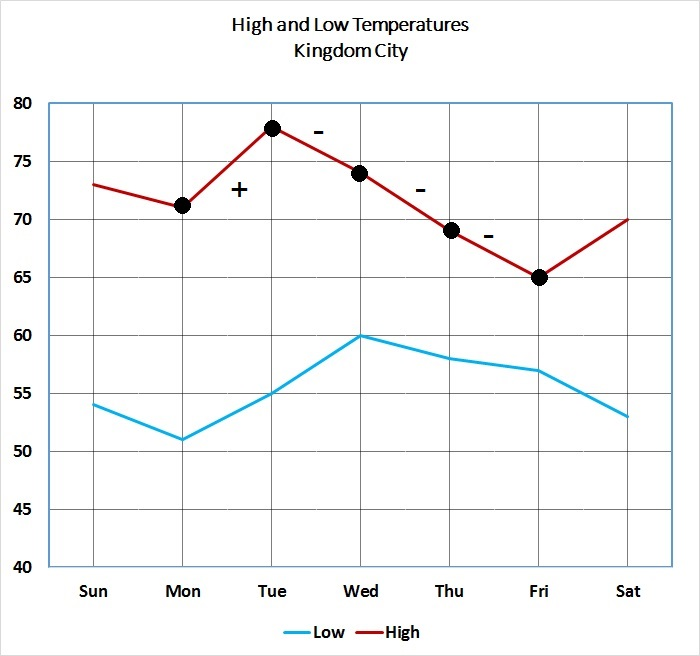Therefore, of the four choices, Monday to Tuesday is correct.

### Example Question #14 : TablesA popular word game uses one hundred tiles, each of which is marked with a letter or a blank. The distribution of the tiles is shown above, with each letter paired with the number of tiles marked with that letter. Notice that there are two blank tiles.

What percent of the vowel tiles are marked with an "A"? (Nearest whole percent).

Note: for purposes of this question, "Y" is a consonant.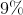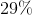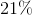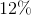Explanation:

There are nine "A" tiles, twelve "E" tiles, nine "I" tiles, eight "O" tiles, and four "U" tiles. This is a total ofvowel tiles.

Out of these tiles, 9 are marked with an "E"; this is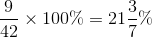.

This rounds to 21%.

### Example Question #21 : Data Analysis And ProbabilityRefer to the above graph, which shows the high and low temperatures for Kingdom City over a one-week period. The temperatures at left are given in degrees Fahrenheit.

Temperatures given in degrees Fahrenheit can be converted to the Celsius scale using the formula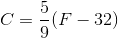Express the highest temperature of the week in degrees Celsius (nearest whole degree).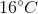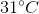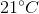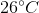Explanation:

As can be seen below, the highest temperature for the week was 78 degrees Fahrenheit, on Tuesday.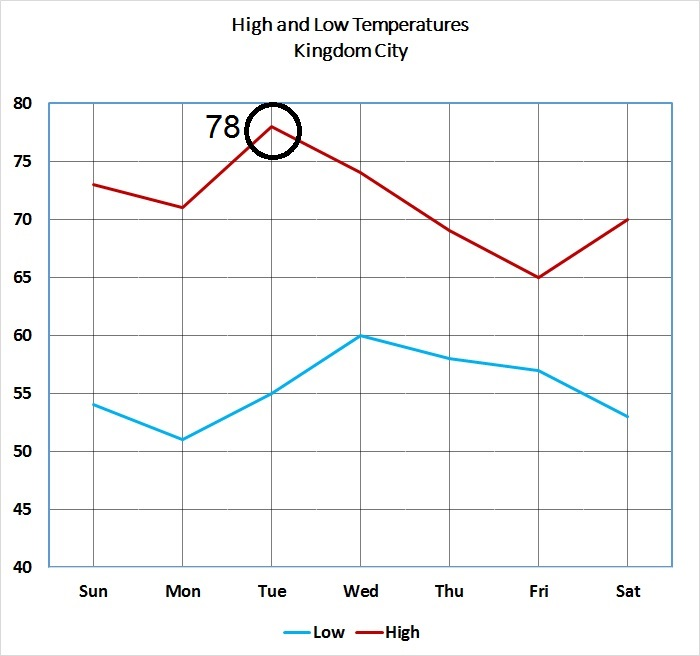To convert this to degrees Celsius, set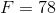and substitute in the given formula: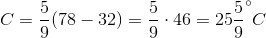. The correct choice is.

### Example Question #22 : Data Analysis And ProbabilityRefer to the above graph, which shows the high and low temperatures for Kingdom City over a one-week period.

The high temperature on Wednesday occurred at 1:00 PM; the low temperature, at the end of the day (midnight). The temperature drop was steady. What was the average drop in temperature per hour between 1:00 PM and midnight (nearest tenth of a second)?

1.3 degrees per hour

2.1 degrees per hour

1.7 degrees per hour

2.5 degrees per hour

1.3 degrees per hour

Explanation:

As can be seen in the diagram below, the high and low temperatures on Wednesday were, respectively, 74 and 60 degrees, for a drop of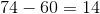degrees.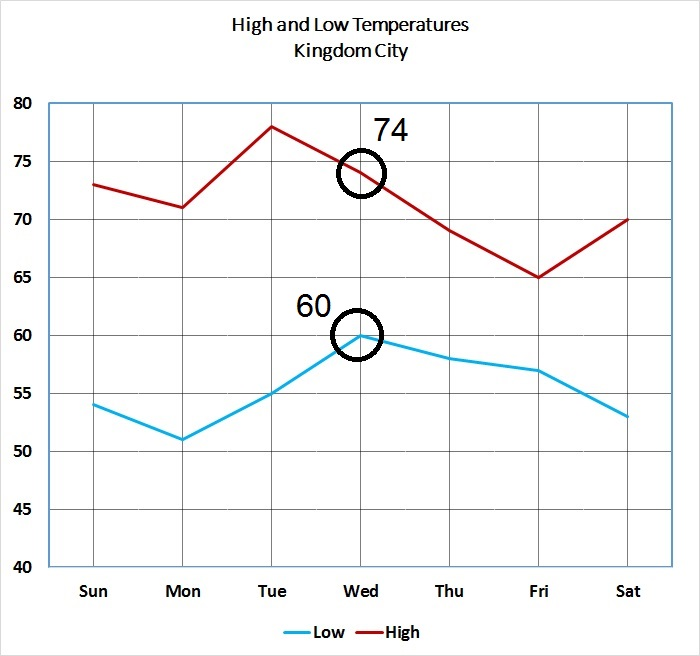This drop occurred over a period of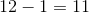hours, for an average drop of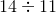degrees per hour. The quotient of this is seen below: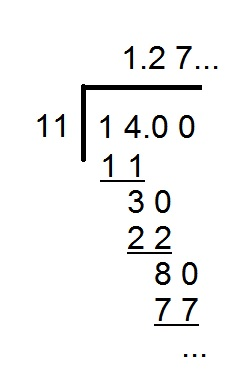This rounds to 1.3 seconds.

### Example Question #23 : Data Analysis And ProbabilityA popular word game uses one hundred tiles, each of which is marked with a letter or a blank. The distribution of the tiles is shown above, with each letter paired with the number of tiles marked with that letter. Notice that there are two blank tiles.

All of the consonant tiles are placed in a box. It is desired to place just enough of the vowel tiles so that the probability of drawing a vowel is. How many vowels must be placed in the box?

Note: for purposes of this question, "Y" is a consonant.Explanation:

There are nine "A" tiles, twelve "E" tiles, nine "I" tiles, eight "O" tiles, and four "U" tiles. This is a total ofThere are also two blanks, so the number of tiles marked with consonants is.

For the probability of drawing a vowel tile to be, the ratio of consonant tiles to vowel tiles must be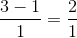- that is, 2 to 1.

If we letstand for the number of vowel tiles to be added, then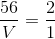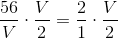vowel tiles.

### Example Question #24 : Data Analysis And Probability

In a class ofstudents, a poll was taken to see how many siblings students had. The results in the poll were then made into a table.

 Number of Siblings Number of Students with the Specific Number of Siblings 0 5 1 9 2 4 3 2

What is the mode for the number of siblings for this class?For this class,students only havesibling. Thus,appears the most often in this data set.